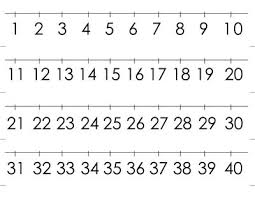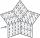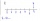# 7 digit number

If 3c54d10 is divisible by 330, what is the sum of c and d?

s1 =  8
s2 =  5
s3 =  11

### Step-by-step explanation:Did you find an error or inaccuracy? Feel free to write us. Thank you!Tips to related online calculators
Do you want to perform natural numbers division - find the quotient and remainder?

## Related math problems and questions:

• Divisible by 5How many three-digit odd numbers divisible by 5, which are in place ten's number 3?
• Five-digit numberAnna thinks of a five-digit number that is not divisible by three or four. If he increments each digit by one, it gets a five-digit number that is divisible by three. If he reduces each digit by one, he gets a five-digit number divisible by four. If it sw
• What are 3What are the two digits which when inserted in the blank spaces will make 234 _ _ divisible by 8?
• Divisible by nineHow many three-digit natural numbers in total are divisible without a remainder by the number 9?
• Twenty-fiveHow many are three-digit natural numbers divisible by 25?
• Four-digit numberFind also a four-digit number, which quadrupled written backwards is the same number.
• Reminder and quotientThere are given numbers A = 135, B = 315. Find the smallest natural number R greater than 1 so that the proportions R:A, R:B are with the remainder 1.
• DivisibilityWrite all the integers x divisible by seven and eight simultaneously, for which the following applies: 100 < x < 200.
• Digit sumDetermine for how many integers greater than 900 and less than 1,001 has digit sum digit of the digit sum number 1.
• Digit sumHow many are three-digit numbers that have a digit sum of 6?
• Prime divisorsFind 2/3 of the sum's ratio and the product of all prime divisors of the number 120.
• Four-digit numbersFind four-digit numbers where all the digits are different. For numbers, the sum of the third and fourth digits is twice the sum of the first two digits, and the sum of the first and fourth digits is equal to the sum of the second and third digits. The di
• PIN codePIN on Michael credit card is a four-digit number. Michael told this to his friend: • It is a prime number - that is, a number greater than 1, which is only divisible by number one and by itself. • The first digit is larger than the second. • The second d
• Air draftThe numbers 1,2,3,4,5 are written on five tickets on the table. Air draft randomly shuffled the tickets and composed a 5-digit number from them. What is the probability that he passed: and, the largest possible number b, the smallest possible number c, a
• Digit sumFind the smallest natural number n, the digit sum of which is equal to 37.
• Digit sumThe digit sum of the two-digit number is nine. When we turn figures and multiply by the original two-digit number, we get the number 2430. What is the original two-digit number?
• NumbersDetermine the number of all positive integers less than 4183444 if each is divisible by 29, 7, 17. What is its sum?